• No products in the cart.

# 204.6.1 Introduction to SVM

##### Getting our basics clear before jumping into SVM.

In the series 204.7 of blogs we will cover SVM or Support Vector Machine.

The first post is about basics of classification and slowly we will lean into SVM with full throttle.

### Introduction

• SVM is another black box method in Machine Learning space.
• Compared to other ml algorithms, SVM totally a different approach to learning.
• The in-depth theory and mathematics of SVM needs great knowledge in vector algebra and numerical analysis.
• We will try to learn the basic principal, philosophy, implementation of SVM.
• SVM was first introduced by Vapnik and Chervonenkis.
• Neural networks try to reduce the squared error and often suffer from overfitting.
• SVM algorithm has better generalization ability. There are many applications where SVM works better than neural networks.

### The Classifier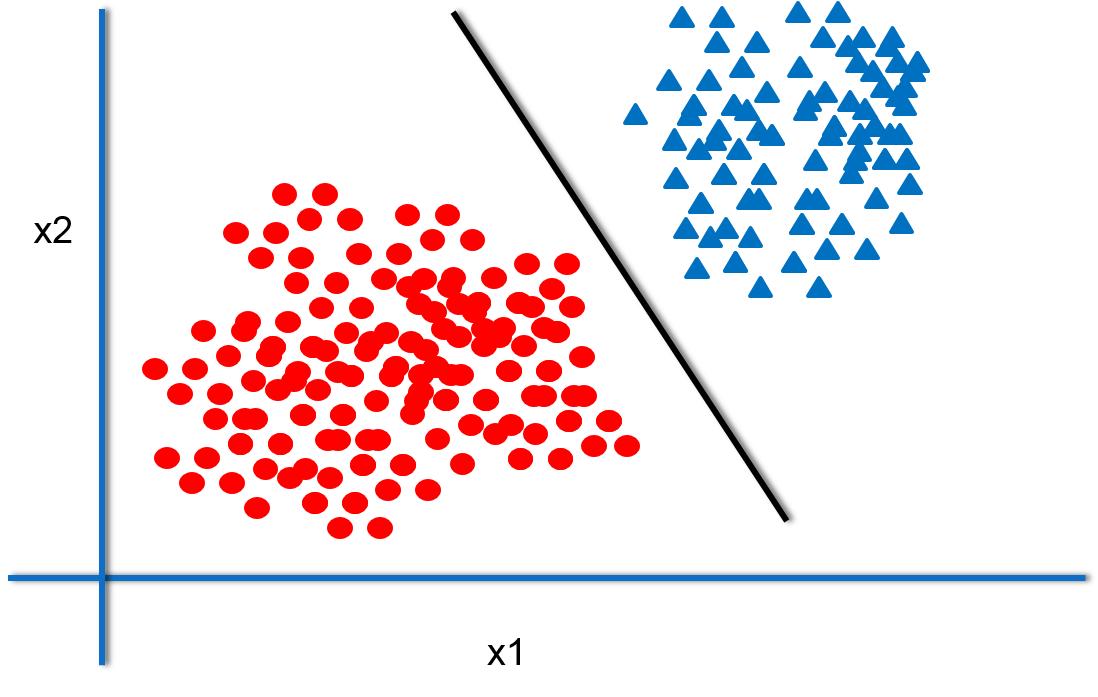• To understand the SVM algorithm easily, we will start with the decision boundary.
• The line or margin that separates the classes.
• Classification, algorithms are all about finding the decision boundaries.
• A good classifier is the one that generalizes well. It should work well on both training and testing data.
• It need not be a straight line always.

### Many Classifiers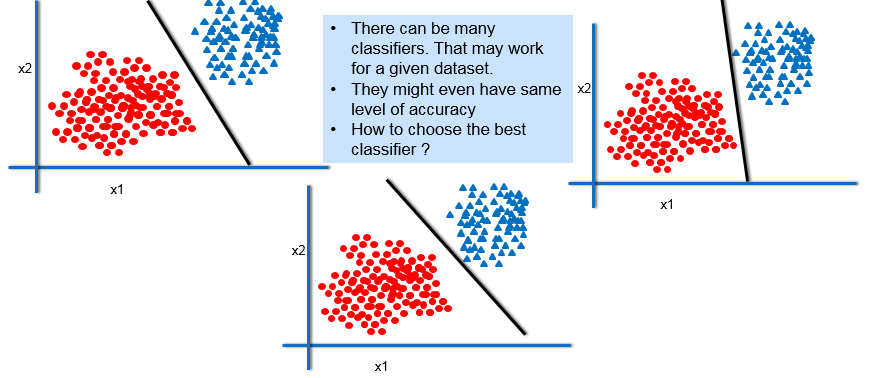### The Margin of Classifier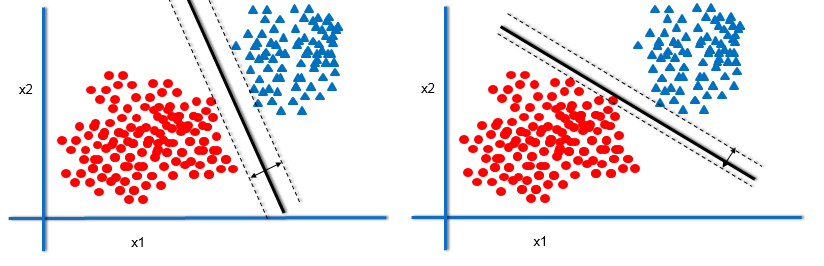Out of all the classifiers, the one that has maximum margin will generalize well. But why?

### The Best Decision Boundary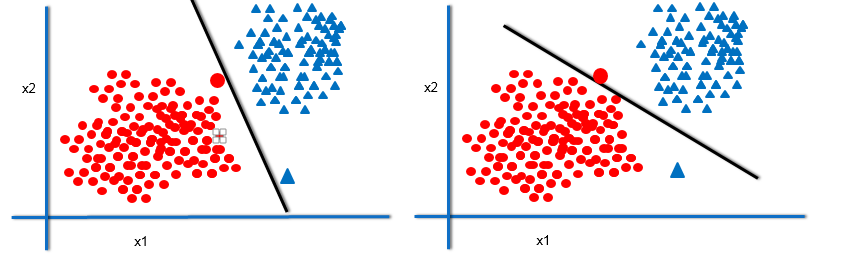• Imagine two more data points. The classifier with maximum margin will be able to classify them more accurately.

### The Maximum Margin Classifier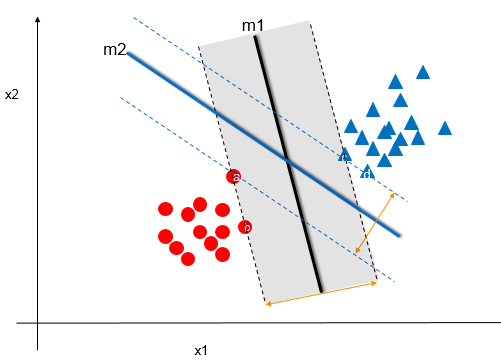• So, the best classifier has maximum margin.
• The classifier that maximizes the distance between itself and the nearest training data.
• In our example a,b,c are the training data points that are near to m1, and a,c,d are the training examples that are near to model m2.
• The model m1 has maximum margin.
• The model m1 works well with the unseen examples.
• The model m1 does good generalization.
• For a given dataset, if we can find a classifier that has maximum margin, then it will assure maximum accuracy.

The next post is about a practice session on a simple classifier.

Link to the next post : https://statinfer.com/204-6-2-practice-simple-classifier/

21st June 2017
0 responses on "204.6.1 Introduction to SVM"